# Figures and Photos From Understanding and Testing Baluns from Nov 2009 AR Magazine.

As sometimes it is a bit hard to see the pictures etc. in AR I reproduce here the various diagrams etc. in a form ( and Colour) that might be more easily seen.

Paul.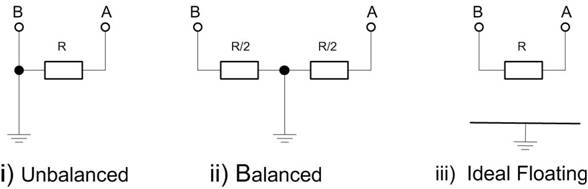Figure 1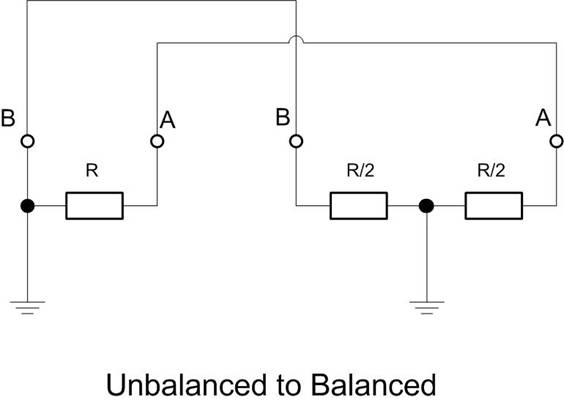Figure 2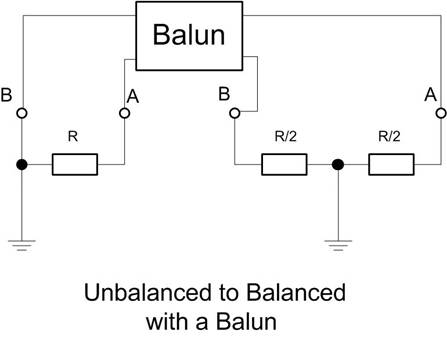Figure 3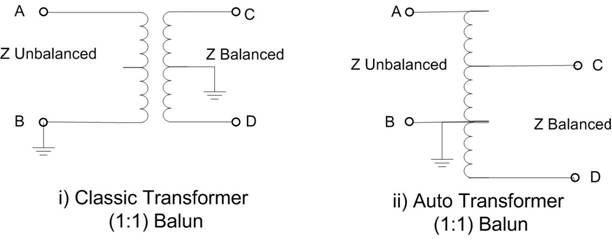Figure 4.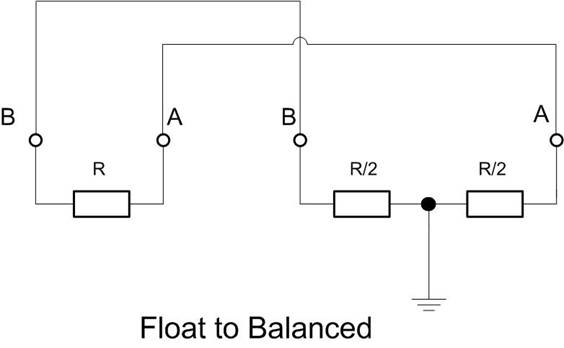Figure 5.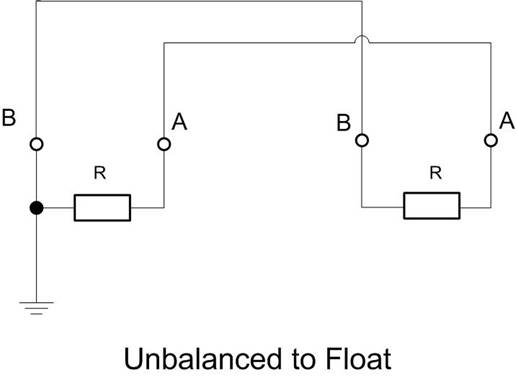Figure 6.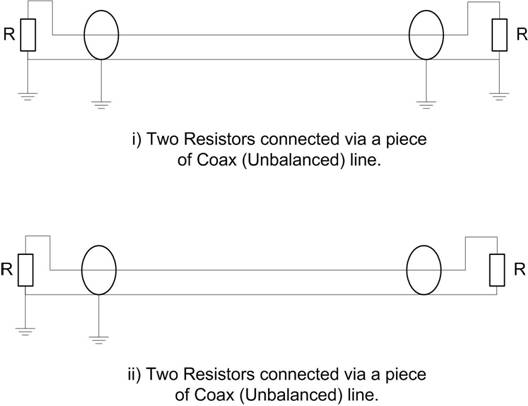Figure 7.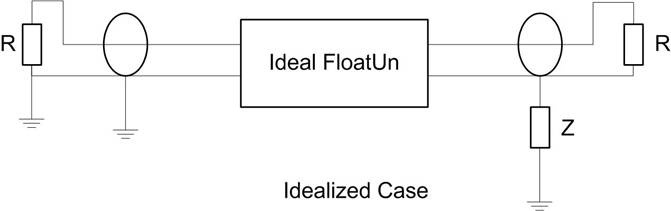Figure 8.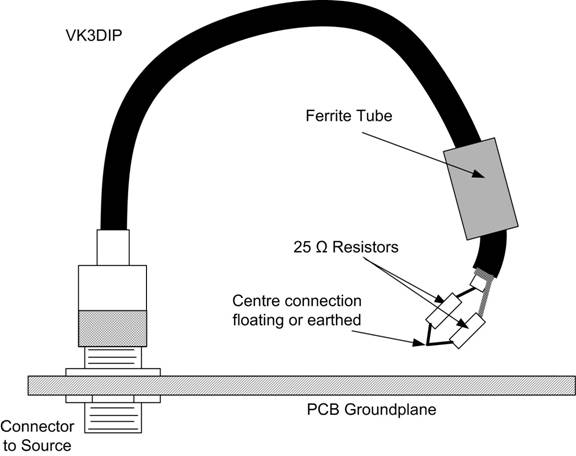Figure 9.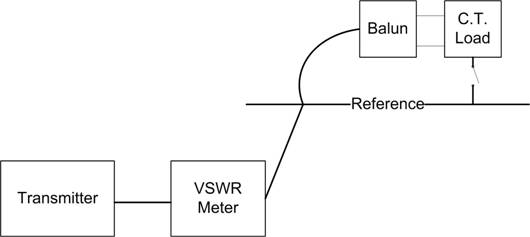Figure 10.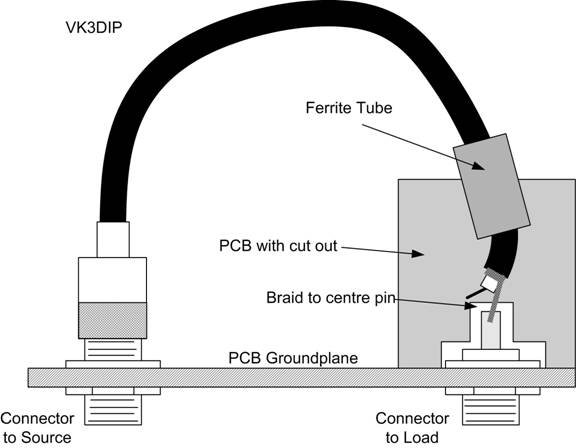Figure 11.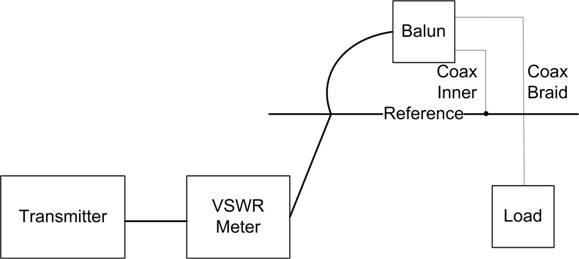Figure 12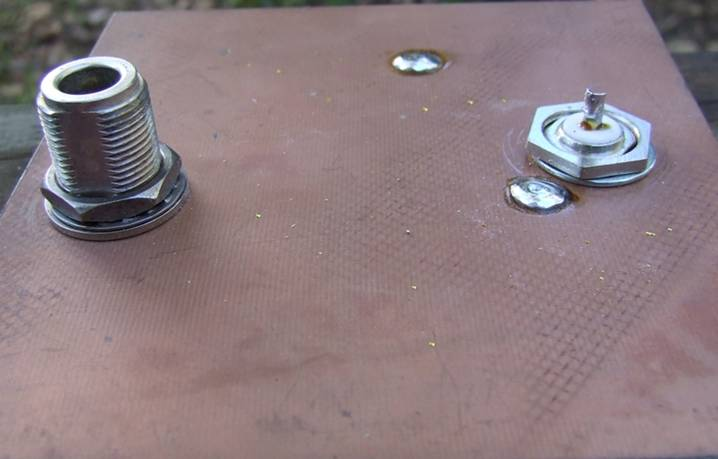Photo1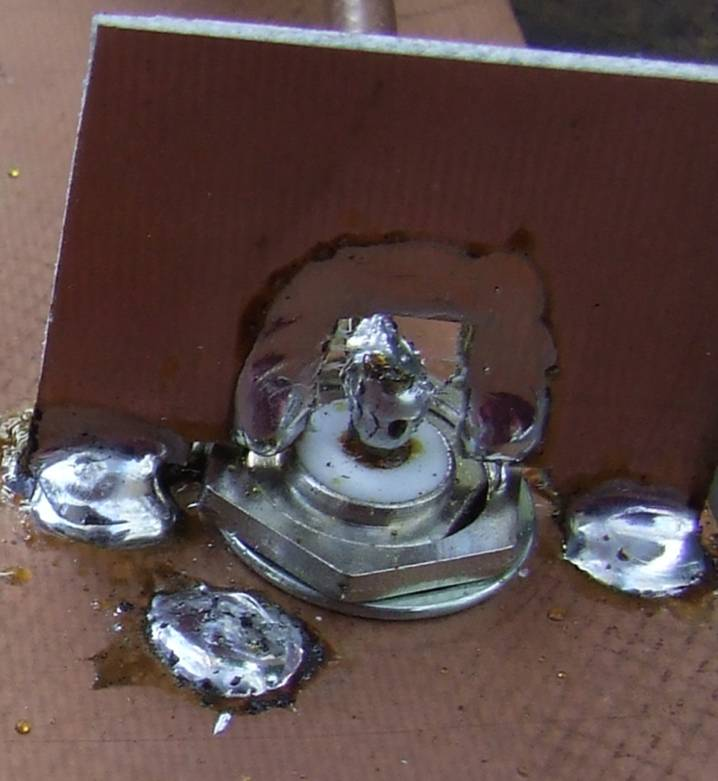Photo 2

Projects

Home